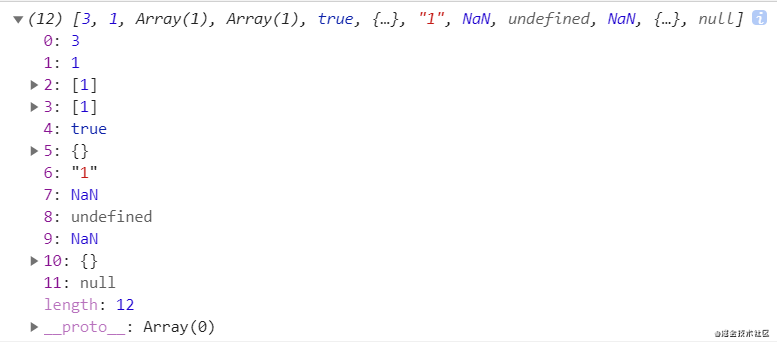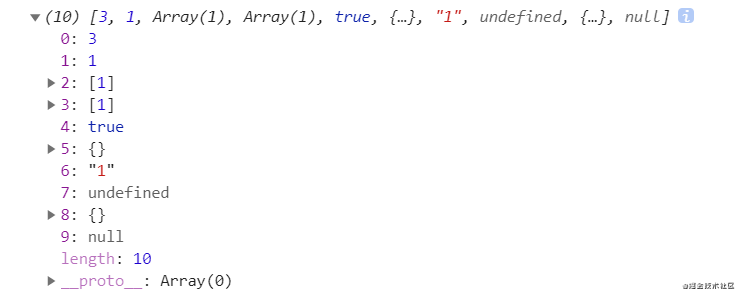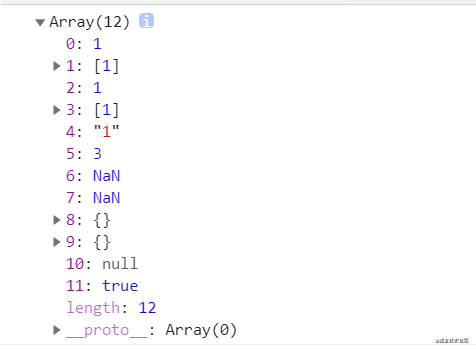# 9 methods of JS array de duplication (including duplicate Nan and complex array types)

2020-11-10 18:08:29

In fact, there are a lot of them on the Internet js Array de duplication method , But I read a lot and I passed code validation myself , There are some flaws in it , So after studying multiple code , I summed up 9 Methods , If there is any mistake, please correct me in time ~
Please indicate the source of the reprint

## Test code

``let arr1 = [3, 1, , 1, , true, true, {}, '1', NaN, undefined, NaN, undefined, {}, null, null];	let arr2 = [];for (let i = 0; i < 100000; i++) { arr2.push(0 + Math.floor((100000 - 0 + 1) * Math.random()));}//  Packaged in Array The prototype object of is better ,this Is the array that calls the method Array.prototype.unique = function () { //...}console.log(arr1.unique());	//  Test the effect of de duplication console.time('test');console.log(arr2.unique());	//  Test de duplication time console.timeEnd('test');``

remarks ：

• `arr1`
• Contains two identical arrays 、 And the array  and 1 The order of （ This is to stand out sort The disadvantages of
• There are two NaN、 Two undefined、 Two null wait , Write down all the data types that you usually use
• There are two identical empty objects {}
• `arr2`
• Put in 10 Ten thousand random pure numbers , Big data can see the difference in time
• about pure number Array of , All of the following methods （ Including various methods on the Internet ） It's all possible
• Correct de duplication `arr1``[3, 1, , true, {}, '1', NaN, undefined, null]`,length = 9.

## One 、 Can't get rid of duplication correctly NaN and Object Methods

### 1. Two kinds of for loop + splice（ Longest time ）

``Array.prototype.unique = function () { for (let i = 0; i < this.length; i++) {  for (let j = i + 1; j < this.length; j++) {   if (this[i] === this[j]) {    this.splice(j, 1);    j--;   }  } } return this;}``• test: 21208.31396484375 ms（ It took 21s....）

You can't get rid of it NaN And complex array types （ such as Object and Array ）

### 2. forEach + indexOf

``Array.prototype.unique = function () { let newArr = []; this.forEach((item) => {  if (newArr.indexOf(item) === -1) {   newArr.push(item);  } }) return newArr;}``

The same as above , I suggest you run it yourself （ I don't want to take a screenshot

• test: 4104.52294921875 ms

You can't get rid of repetitive NaN And complex data types

• reason ：indexOf Think NaN It's not equal to NaN

### 3. filter + indexOf

``Array.prototype.unique = function () { return this.filter((item, index) => {  //  utilize indexOf Check whether the first position of an element in the array is equal to the current position of the element , If not, it means that the element is a repeating element   return this.indexOf(item) === index; })}``• test: 5682.358154296875 ms

You cannot remove duplicate complex data types , At the same time, all the NaN

• reason ：indexOf Think NaN It's not equal to NaN , So they are not considered repetitive elements .

### 4. for + sort（sort There is a problem ）

``Array.prototype.unique = function () { let newArr = []; this.sort(); for (let i = 0; i < this.length; i++) {  if (this[i] !== this[i + 1]) {   newArr.push(this[i]);  } } return newArr;}``• test: 61.96484375 ms

belt sort The method is only right for pure number Or pure string type It works , It can't distinguish 1 and '1', Because it is converting elements into strings , Then compare their UTF-16 Code unit value sequence is built .

If there is a sort like this [1, '1', 1], Then use the method of comparison before and after to go back to heavy will appear problem

Here he'll put undefined And remove all of them , as a result of `sort()` After sorting the methods ,`undefined` At the end of the line , And the last one `undefined` Want to be with `this[length]` Compare , And this value doesn't exist , And it is undefined, They will be considered to be the same value .